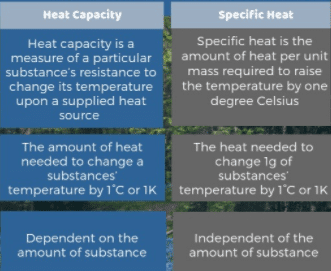# What is Difference between specific heat and Heat capacity?Specific heat is the heat that must be supplied to the unit of mass of a given substance to raise its temperature by one degree and it is an intensive property and the heat capacity depends on the amount of matter considered, therefore it is extensive.

## Specific Heat Capacity definition

The specific heat of a substance is the amount of heat required to raise the temperature of 1 kg mass of that substance through 1 K.

### Specific heat formula

Generally, when a body is heated, its temperature increases. An increase in the temperature of a body is found to be proportional to the amount of heat absorbed by it. It has also been observed that the quantity of heat ΔQ required to raise the temperature Δ T of a body is proportional to the mass of the body. Thus

Δ Q ∝ m Δ T

or     Δ Q = c m Δ T ………….(1)

Here Δ Q is the amount of heat absorbed by the body and c is the constant of proportionality called the heat capacity of simple specific heat.

Read also: Latent heat of fusion and vaporization

### Specific heat capacity formulaIn SI units, mass m is measured in kilogram (kg), heat Δ Q is measured in Joule (J) and temperature increase Δ T is taken in kelvin (K). Hence, the SI unit of specific heat bis Jkg-1 K-1.

### Examples of Specific heat

Specific heats of some common substances are given in the table:### What is the Specific heat capacity of water?

The specific heat of water is 4200 J Kg-1K-1 and that of dry soil is about 810 J Kg-1K-1. As a result, the temperature of soil would increase five times more than the same mass of water by the same amount of heat. Thus, the temperature of land rises and falls more rapidly than that of the sea. Hence, the temperature variations from summer to winter are much smaller at places near the sea than land far away from the sea.

Water has a large specific heat capacity. For this reason, it is very useful in storing and carrying thermal energy due to its high specific heat capacity. The cooling system of automobiles uses water to carry away unwanted thermal energy.

In an automobile, a large amount of heat is produced by its engine due to which its temperature goes on increasing. The engine would cease unless it is not cooled down. Water circulating around the engine by arrows maintains its temperature. Water absorbs unwanted thermal energy of the engine and dissipates heat through its radiator.

In central heating systems such as hot water is used to carry thermal energy through pipes from the boiler to radiators. These radiators are fixed inside the house at suitable places.

### Heat Capacity definition

The heat capacity of a body is the quantity of thermal energy absorbed by it for one kelvin (1 K) increase in its temperature.

Thus, if the temperature of a body increase through Δ T on adding  Q amount of heat, then its heat capacity will be ΔQ/ Δ T. Putting the value of Δ Q, we get:

Heat capacity = Δ Q/ Δ T = m c Δ T/ Δ T

heat capacity = m c ………..(3)

Equation (3) show that the heat capacity of a body is equal to the product of its mass of the body and its specific heat capacity. For example, the heat capacity of 5 kg of water is ( 5 kg × 4200 J kg -1 -1) 21000 JK -1. That is; 5 kg of water needs 21000 Joules of heat for every 1 K rise in its temperature. Thus, the larger is the quantity of a substance, the large will be its heat capacity.

#### Change of State:

Matter can be changed from one state to another. For such a change to occur, thermal energy is added to or removed from a substance.Watch also: